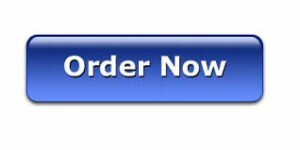# Cash Flow Statement Assignment

## Cash Flow Statement Assignment

Develop an income statement and cash flow statement for 2012, based on the data provided below for the year 2012. All sales are collected when the sale is made and all expenses are paid when the expense incurred.a. Income statement data for 2012

1. Units produced and sold = 420

2. Sales ( \$80 per unit selling price) = \$ 33600

3. cost of goods sold ( \$30 per unit, all variable costs) = \$ 12600

4. labor = \$0

6. Bank fees = \$150

7. phone/internet = \$1200

8. Shipping(\$3 per unit) = \$1260

9. utilities = \$900

10. office supplies = \$800

11. interest expense on note payable = \$350

12. Depreciation( straight line) = \$800

13. Income tax rate = 26%

b. Other financial data for 2012:

1. Proceeds from the sale of equipment = \$3000. The equipment originally cost \$1000 and had accumulated depreciation of \$200.

2. Purchase of equipment = \$1600 ( The machine is purchased on the last day of 2012 so no depreciation expense is recorded)

3. Repayment of note payable = \$5000

4. Consider any data relevant from the income statement. Get Accounting homework help today